The concept of continuously compounded interest rate can be explained by taking an example:

Say, an investment of \$100 today is giving a return of \$120 in the next year. So, the rate of return is 20%. If the interest is paid twice every year, and the rate of interest remains constant, and the amount earned at the end of the year is \$120, the rate of interest can be given as: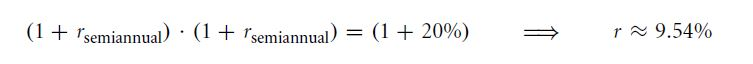If the semi-annual interest rate is multiplied by 2, r becomes 19.08%. If you receive interest 12 times a year, then the rate of interest will be: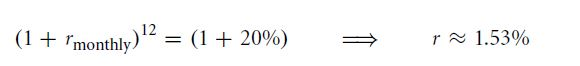If we multiply the monthly rate of interest by 12, r becomes 18.36%. Now, if you receive interest 365 times in a year, then the rate of interest will be: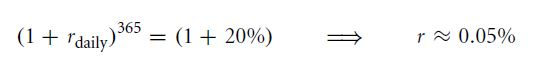Multiply this daily rate of interest by 365 and r will be 18.25%.

The continuously compounded interest rate can be calculated by taking natural logarithm of 1 plus the simple rate of interest.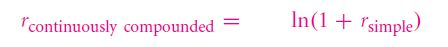Similarly, the simple rate of interest can also be calculated from the above formula: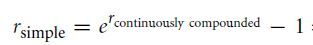Continuously compounded interests can be added. These interest rates are symmetric. The range of continuously compounded interest rate lie between -∞ to +∞. Compounded interest rates are used in Black-Scholes option formula.

REVIEW QUESTIONS

1. Calculate the continuously compounded interest rate if a bond pays \$150 for every investment of \$100.
2. If a bond pays an interest rate of 10% compounded continuously, the money received after maturity is reinvested 20% interest rate, which is also compounded continuously. What will the value of a present day investment of \$100? Calculate the simple and continuously compounded rate of interest over the two periods.

Links of Next Financial Accounting Topics:-### Customer Reviews

My Homework Help
Rated 5.0 out of 5 based on 510 customer reviews at